# Chapter 24 Gausss Law Gauss Law Gauss Law

• Slides: 39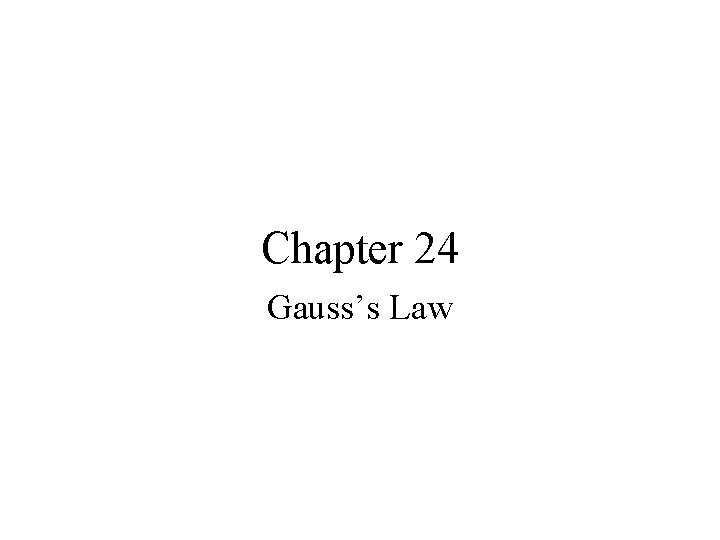Chapter 24 Gauss’s Law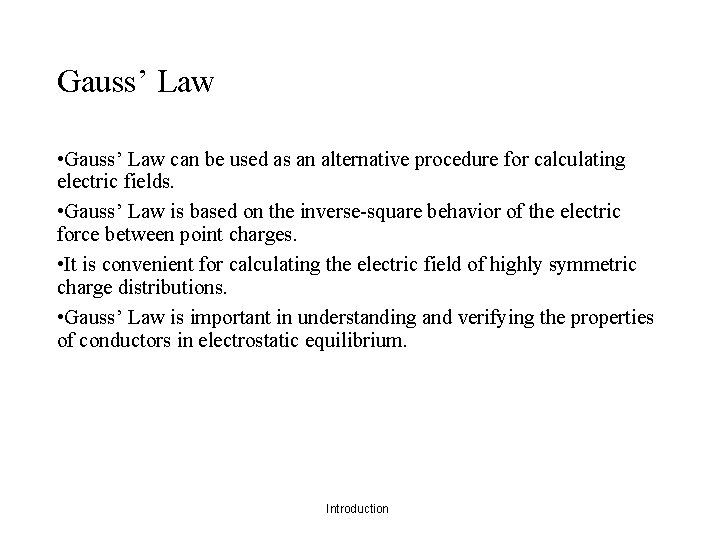Gauss’ Law • Gauss’ Law can be used as an alternative procedure for calculating electric fields. • Gauss’ Law is based on the inverse-square behavior of the electric force between point charges. • It is convenient for calculating the electric field of highly symmetric charge distributions. • Gauss’ Law is important in understanding and verifying the properties of conductors in electrostatic equilibrium. Introduction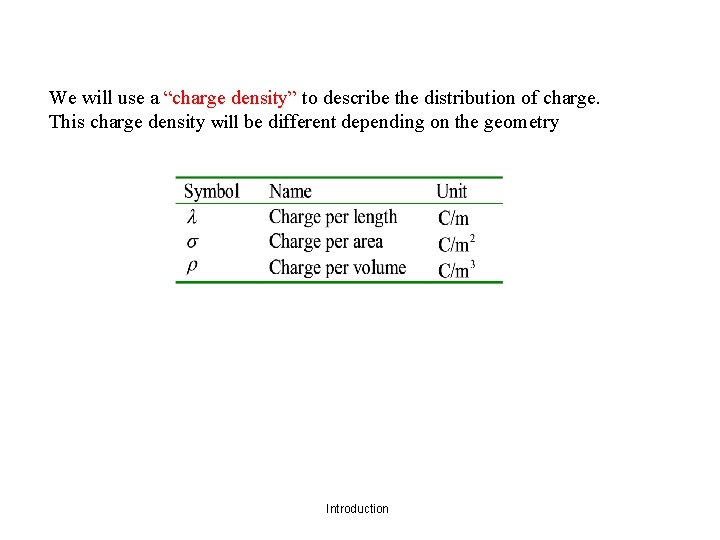We will use a “charge density” to describe the distribution of charge. This charge density will be different depending on the geometry Introduction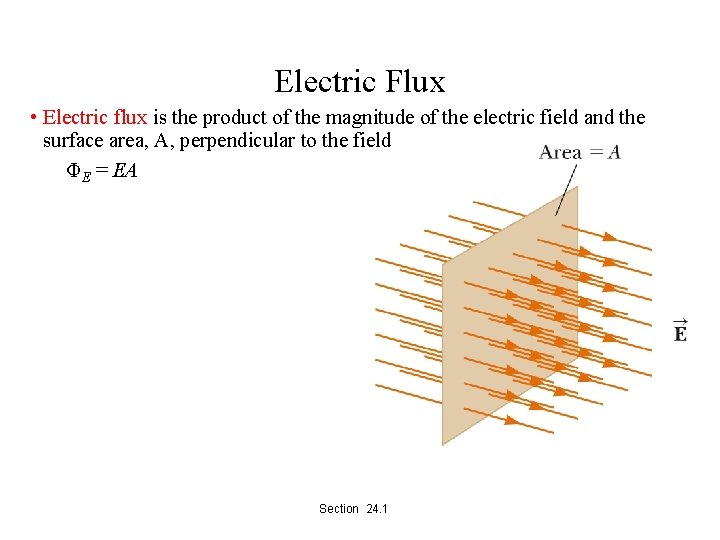Electric Flux • Electric flux is the product of the magnitude of the electric field and the surface area, A, perpendicular to the field ΦE = EA Section 24. 1Electric Flux, General Area • The electric flux is proportional to the • number of electric field lines • penetrating some surface • The field lines may make some • angle θ with the perpendicular to the surface • Then ΦE = EA cos θ • The flux is a maximum when the surface is perpendicular to the field • The flux is a minimum (zero) when the surface is (parallel) to the field • The electric flux is proportional to the number of electric field lines penetrating some surface. • The field lines may make some angle θ with the perpendicular to the surface. Section • Then ΦE = EA cos θ 24. 1Electric Flux, Interpreting the Equation • The flux is a maximum when the surface is perpendicular to the field. • θ = 0° • The flux is zero when the surface is parallel to the field. • θ = 90° • If the field varies over the surface, Φ = EA cos θ is valid for only a small element of the area. Section 24. 1Electric Flux, General • In the more general case, look at a small area element. • In general, this becomes • The surface integral means the integral must be evaluated over the surface in question. • In general, the value of the flux will depend both on the field pattern and on the surface. Section 24. 1Electric Flux, Closed Surface • Assume a closed surface • The vectors point in different directions. • At each point, they are perpendicular to the surface. • By convention, they point outward. Section 24. 1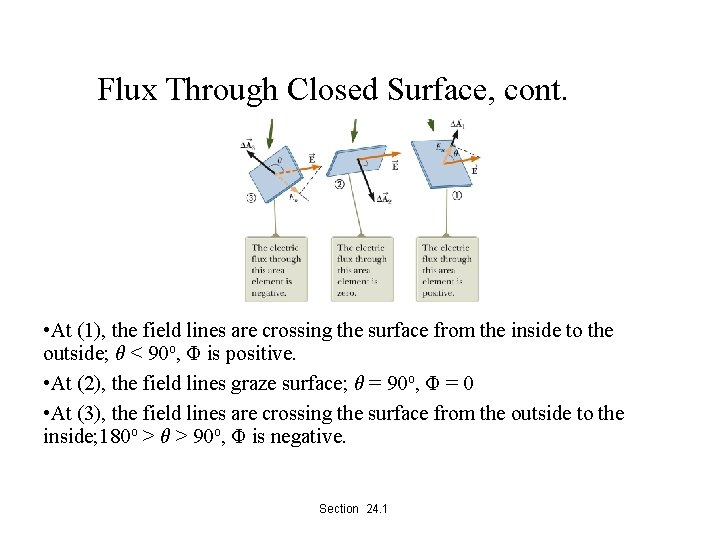Flux Through Closed Surface, cont. • At (1), the field lines are crossing the surface from the inside to the outside; θ < 90 o, Φ is positive. • At (2), the field lines graze surface; θ = 90 o, Φ = 0 • At (3), the field lines are crossing the surface from the outside to the inside; 180 o > θ > 90 o, Φ is negative. Section 24. 1Flux Through Closed Surface, final • The net flux through the surface is proportional to the net number of lines leaving the surface. • This net number of lines is the number of lines leaving the surface minus the number entering the surface. • If En is the component of the field perpendicular to the surface, then • The integral is over a closed surface. Section 24. 1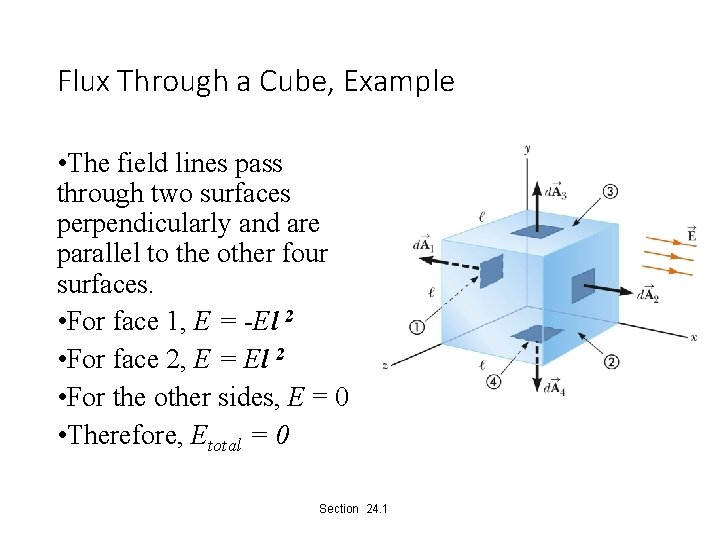Flux Through a Cube, Example • The field lines pass through two surfaces perpendicularly and are parallel to the other four surfaces. • For face 1, E = -El 2 • For face 2, E = El 2 • For the other sides, E = 0 • Therefore, Etotal = 0 Section 24. 1Karl Friedrich Gauss • 1777 – 1855 • Made contributions in • Electromagnetism • Number theory • Statistics • Non-Euclidean geometry • Cometary orbital mechanics • A founder of the German Magnetic Union • Studies the Earth’s magnetic field Section 24. 2Gauss’s Law, Introduction • Gauss’s law is an expression of the general relationship between the net electric flux through a closed surface and the charge enclosed by the surface. • The closed surface is often called a gaussian surface. • Gauss’s law is of fundamental importance in the study of electric fields. Section 24. 2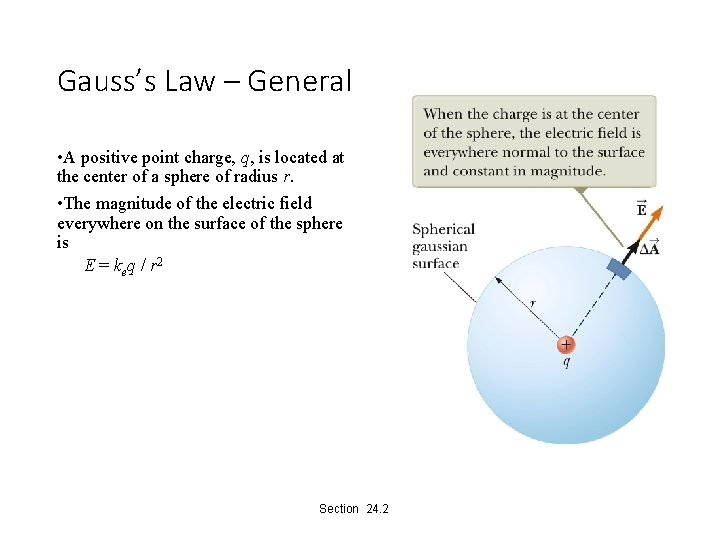Gauss’s Law – General • A positive point charge, q, is located at the center of a sphere of radius r. • The magnitude of the electric field everywhere on the surface of the sphere is E = keq / r 2 Section 24. 2Gauss’s Law – General, cont. • The field lines are directed radially outward and are perpendicular to the surface at every point. • This will be the net flux through the gaussian surface, the sphere of radius r. • We know E = keq/r 2 and Asphere = 4πr 2, Section 24. 2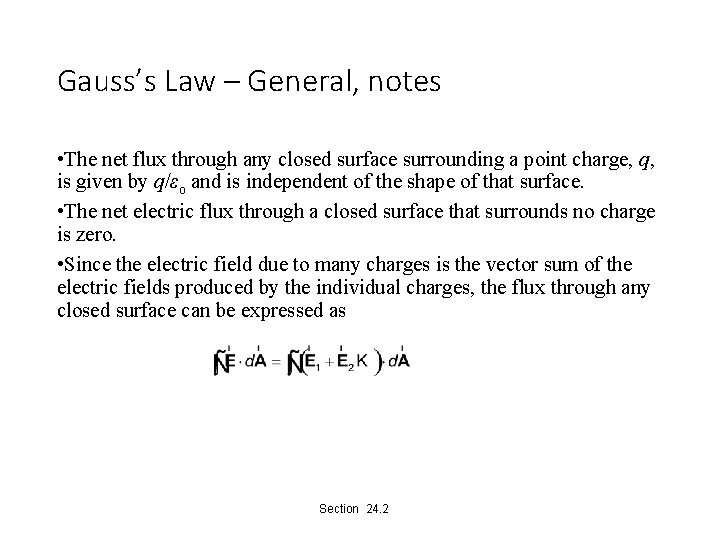Gauss’s Law – General, notes • The net flux through any closed surface surrounding a point charge, q, is given by q/εo and is independent of the shape of that surface. • The net electric flux through a closed surface that surrounds no charge is zero. • Since the electric field due to many charges is the vector sum of the electric fields produced by the individual charges, the flux through any closed surface can be expressed as Section 24. 2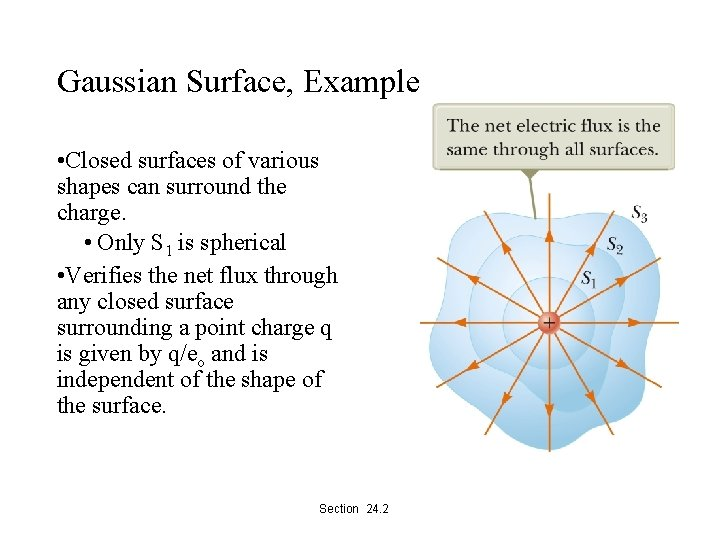Gaussian Surface, Example • Closed surfaces of various shapes can surround the charge. • Only S 1 is spherical • Verifies the net flux through any closed surface surrounding a point charge q is given by q/eo and is independent of the shape of the surface. Section 24. 2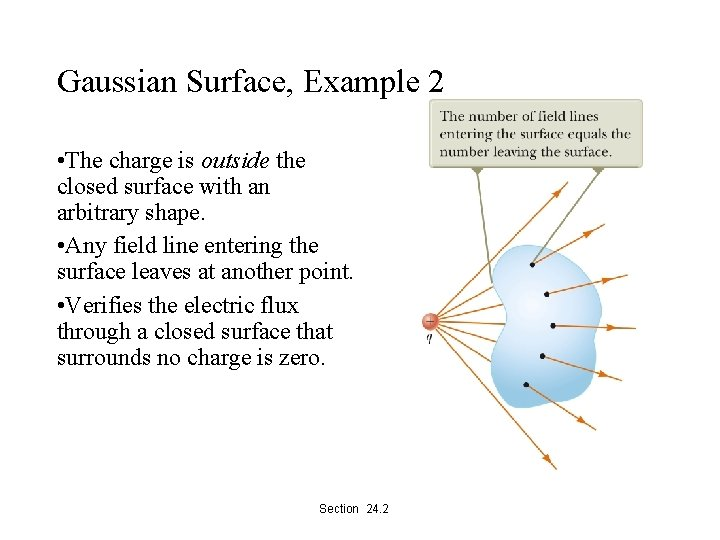Gaussian Surface, Example 2 • The charge is outside the closed surface with an arbitrary shape. • Any field line entering the surface leaves at another point. • Verifies the electric flux through a closed surface that surrounds no charge is zero. Section 24. 2Gauss’s Law – Final • The mathematical form of Gauss’s law states • qin is the net charge inside the surface. • represents the electric field at any point on the surface. • is the total electric field and may have contributions from charges both inside and outside of the surface. • Although Gauss’s law can, in theory, be solved to find for any charge configuration, in practice it is limited to symmetric situations. Section 24. 2Applying Gauss’s Law • To use Gauss’s law, you want to choose a gaussian surface over which the surface integral can be simplified and the electric field determined. • Take advantage of symmetry. • Remember, the gaussian surface is a surface you choose, it does not have to coincide with a real surface. Section 24. 3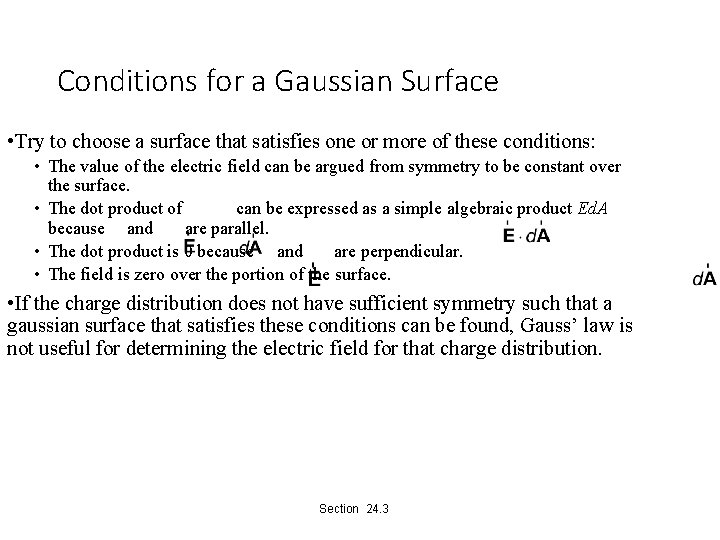Conditions for a Gaussian Surface • Try to choose a surface that satisfies one or more of these conditions: • The value of the electric field can be argued from symmetry to be constant over the surface. • The dot product of can be expressed as a simple algebraic product Ed. A because and are parallel. • The dot product is 0 because and are perpendicular. • The field is zero over the portion of the surface. • If the charge distribution does not have sufficient symmetry such that a gaussian surface that satisfies these conditions can be found, Gauss’ law is not useful for determining the electric field for that charge distribution. Section 24. 3Field Due to a Spherically Symmetric Charge Distribution • Select a sphere as the gaussian surface. • For r >a Section 24. 3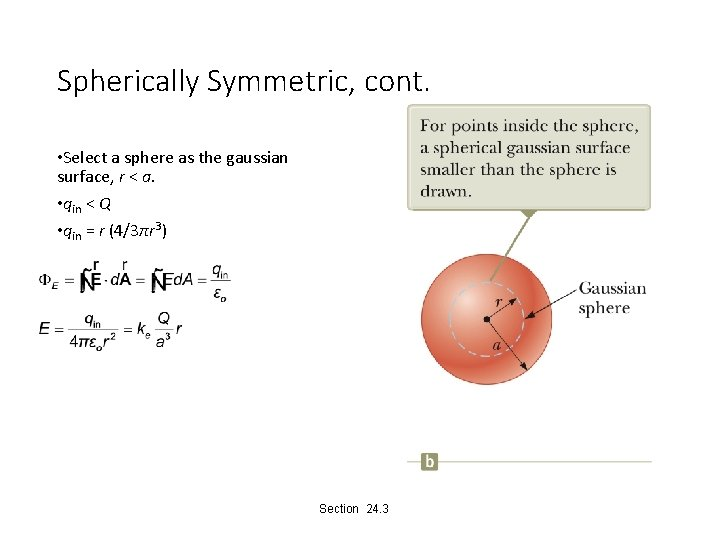Spherically Symmetric, cont. • Select a sphere as the gaussian surface, r < a. • qin < Q • qin = r (4/3πr 3) Section 24. 3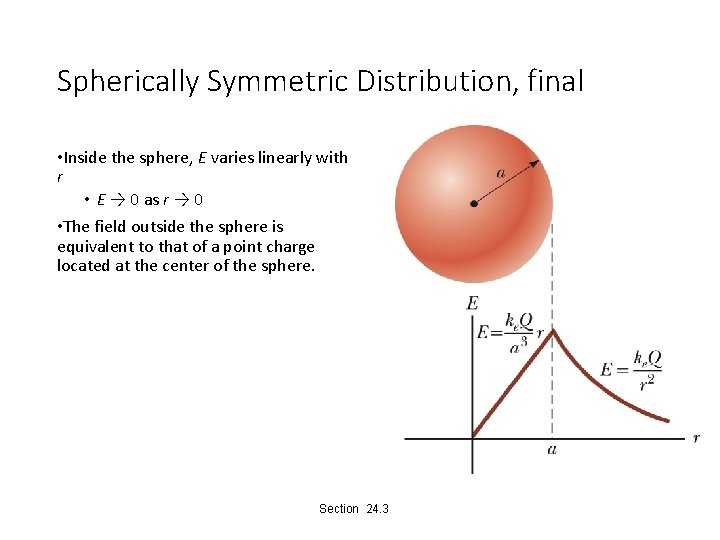Spherically Symmetric Distribution, final • Inside the sphere, E varies linearly with r • E → 0 as r → 0 • The field outside the sphere is equivalent to that of a point charge located at the center of the sphere. Section 24. 3Field at a Distance from a Line of Charge • Select a cylindrical charge distribution. • The cylinder has a radius of r and a length of ℓ. • is constant in magnitude and perpendicular to the surface at every point on the curved part of the surface. • Use Gauss’s law to find the field. Section 24. 3Field Due to a Line of Charge, cont. • The end view confirms the field is perpendicular to the curved surface. • The field through the ends of the cylinder is 0 since the field is parallel to these surfaces. Section 24. 3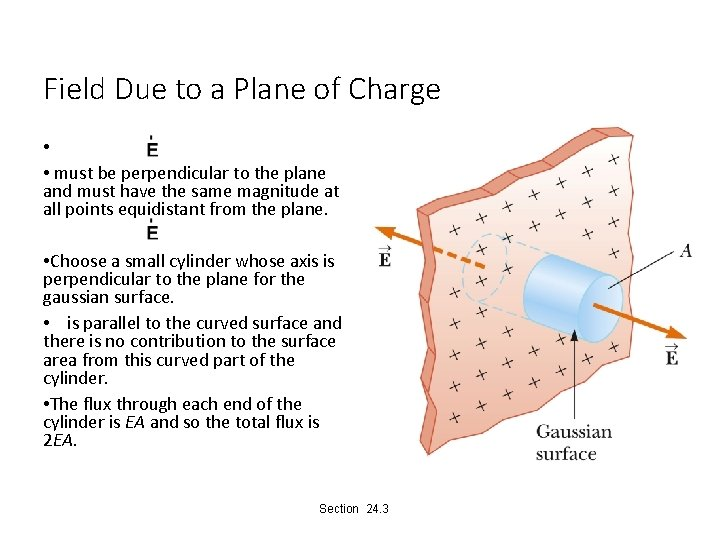Field Due to a Plane of Charge • • must be perpendicular to the plane and must have the same magnitude at all points equidistant from the plane. • Choose a small cylinder whose axis is perpendicular to the plane for the gaussian surface. • is parallel to the curved surface and there is no contribution to the surface area from this curved part of the cylinder. • The flux through each end of the cylinder is EA and so the total flux is 2 EA. Section 24. 3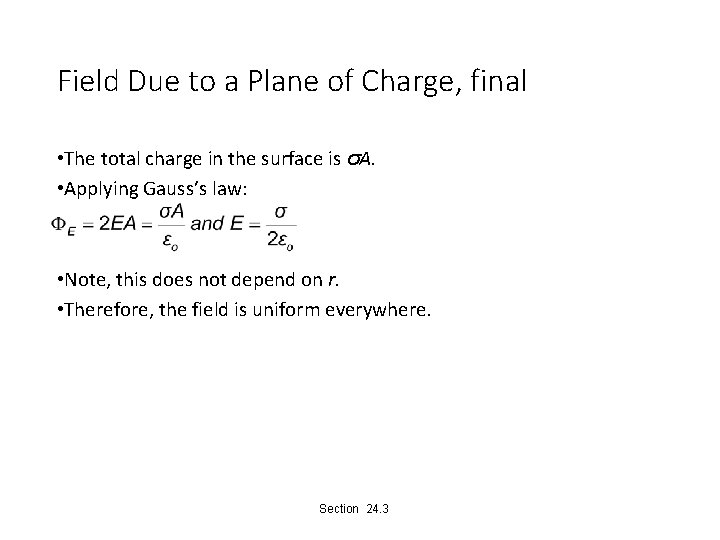Field Due to a Plane of Charge, final • The total charge in the surface is σA. • Applying Gauss’s law: • Note, this does not depend on r. • Therefore, the field is uniform everywhere. Section 24. 3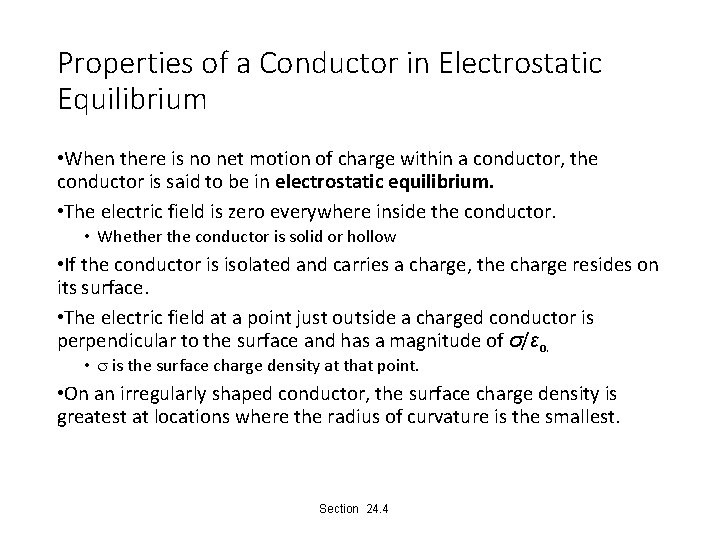Properties of a Conductor in Electrostatic Equilibrium • When there is no net motion of charge within a conductor, the conductor is said to be in electrostatic equilibrium. • The electric field is zero everywhere inside the conductor. • Whether the conductor is solid or hollow • If the conductor is isolated and carries a charge, the charge resides on its surface. • The electric field at a point just outside a charged conductor is perpendicular to the surface and has a magnitude of σ/εo. • s is the surface charge density at that point. • On an irregularly shaped conductor, the surface charge density is greatest at locations where the radius of curvature is the smallest. Section 24. 4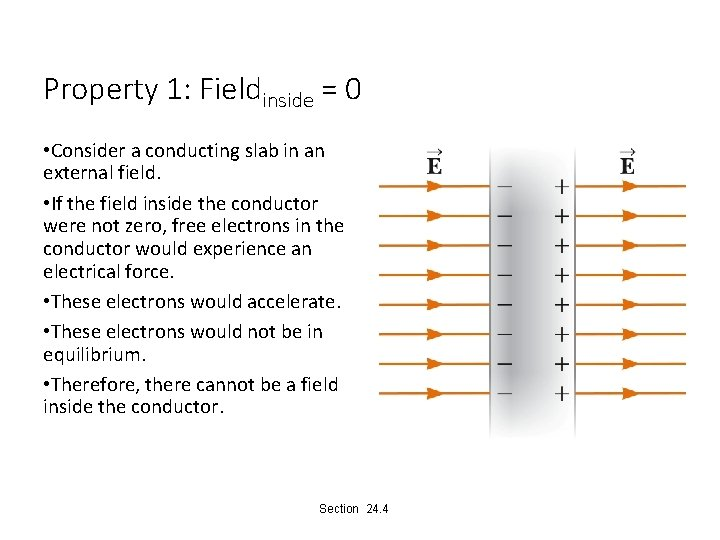Property 1: Fieldinside = 0 • Consider a conducting slab in an external field. • If the field inside the conductor were not zero, free electrons in the conductor would experience an electrical force. • These electrons would accelerate. • These electrons would not be in equilibrium. • Therefore, there cannot be a field inside the conductor. Section 24. 4Property 1: Fieldinside = 0, cont. • Before the external field is applied, free electrons are distributed throughout the conductor. • When the external field is applied, the electrons redistribute until the magnitude of the internal field equals the magnitude of the external field. • There is a net field of zero inside the conductor. • This redistribution takes about 10 -16 s and can be considered instantaneous. • If the conductor is hollow, the electric field inside the conductor is also zero. • Either the points in the conductor or in the cavity within the conductor can be considered. Section 24. 4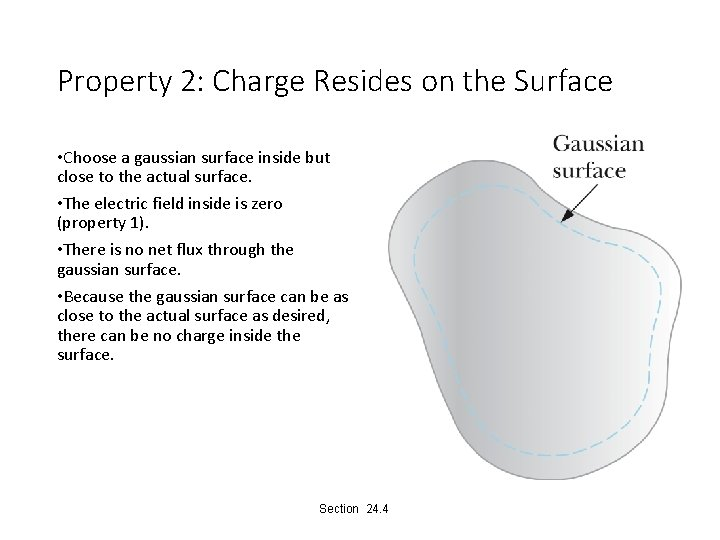Property 2: Charge Resides on the Surface • Choose a gaussian surface inside but close to the actual surface. • The electric field inside is zero (property 1). • There is no net flux through the gaussian surface. • Because the gaussian surface can be as close to the actual surface as desired, there can be no charge inside the surface. Section 24. 4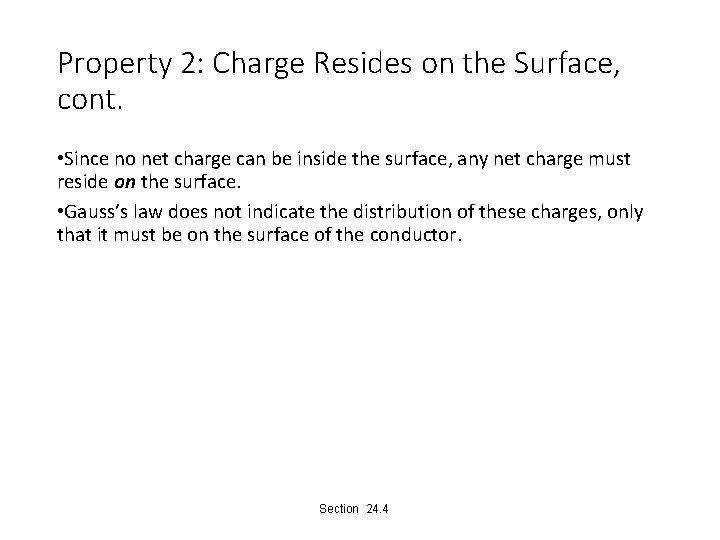Property 2: Charge Resides on the Surface, cont. • Since no net charge can be inside the surface, any net charge must reside on the surface. • Gauss’s law does not indicate the distribution of these charges, only that it must be on the surface of the conductor. Section 24. 4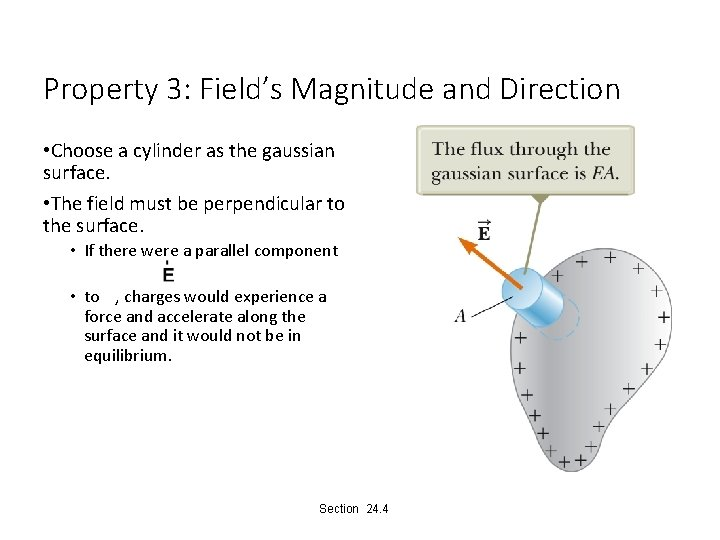Property 3: Field’s Magnitude and Direction • Choose a cylinder as the gaussian surface. • The field must be perpendicular to the surface. • If there were a parallel component • to , charges would experience a force and accelerate along the surface and it would not be in equilibrium. Section 24. 4Property 3: Field’s Magnitude and Direction, cont. • The net flux through the gaussian surface is through only the flat face outside the conductor. • The field here is perpendicular to the surface. • Applying Gauss’s law Section 24. 4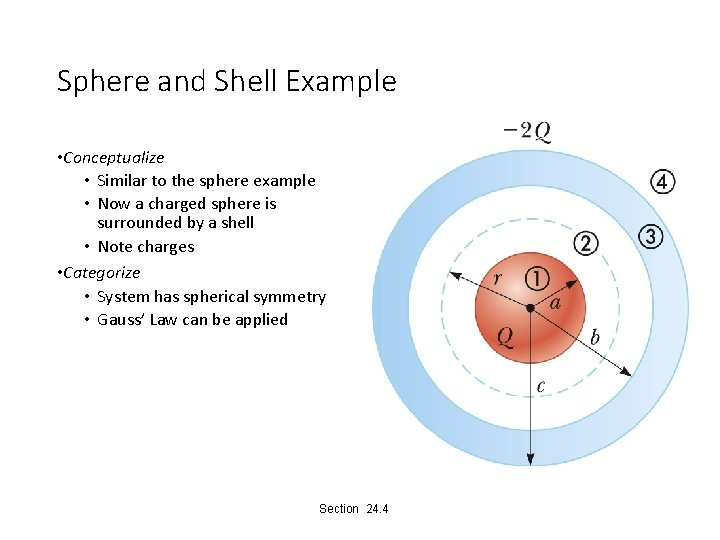Sphere and Shell Example • Conceptualize • Similar to the sphere example • Now a charged sphere is surrounded by a shell • Note charges • Categorize • System has spherical symmetry • Gauss’ Law can be applied Section 24. 4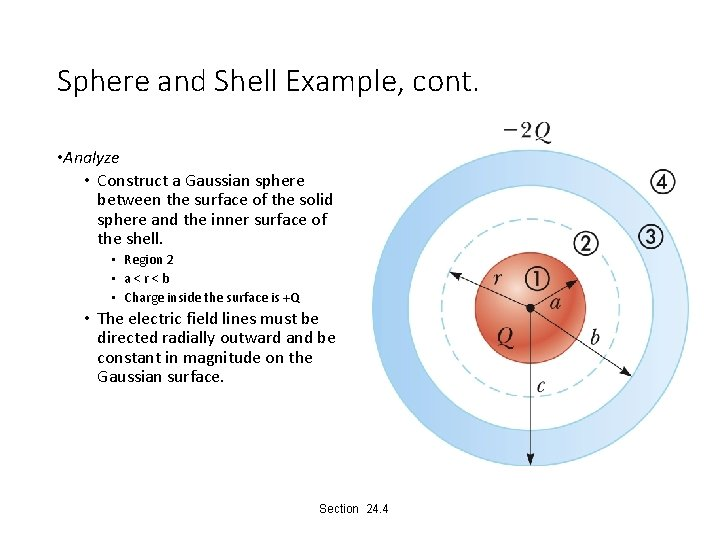Sphere and Shell Example, cont. • Analyze • Construct a Gaussian sphere between the surface of the solid sphere and the inner surface of the shell. • Region 2 • a<r<b • Charge inside the surface is +Q • The electric field lines must be directed radially outward and be constant in magnitude on the Gaussian surface. Section 24. 4Sphere and Shell Example, 3 • Analyze, cont. • The electric field for each area can be calculated. Section 24. 4Sphere and Shell Example • Finalize • Check the net charge. • Think about other possible combinations. • What if the sphere were conducting instead of insulating? Section 24. 4